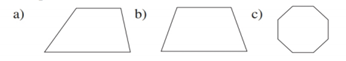Chapter 2.6, Problem 8EElementary Geometry For College St...

7th Edition
Alexander + 2 others
ISBN: 9781337614085

Solutions

Chapter
SectionElementary Geometry For College St...

7th Edition
Alexander + 2 others
ISBN: 9781337614085
Textbook Problem

Which geometric figures have symmetry with respect to a point?To determine

To choose:

The geometric figures having symmetry with respect to a point.

Explanation

Consider the geometric figure (a).

Figure (1)

Consider arbitrary points A on different point on the polygon.

Figure (2)

Since, there is no point of symmetry exists for the quadrilateral as it is irregular in shape.

The geometric figure (a) is not symmetric about point P.

Consider the geometric figure (b) and consider point P at the centre of the geometric figure with an arbitrary point A

Figure (3)

The geometric figure (b) is not symmetric about point P as the horizontal centre and vertical centre do not coincides for a trapezoid. There is no point of symmetry exists for the trapezoid.

Consider the geometric figure (c) and consider point P at the centre of the geometric figure.

Still sussing out bartleby?

Check out a sample textbook solution.

See a sample solution

The Solution to Your Study Problems

Bartleby provides explanations to thousands of textbook problems written by our experts, many with advanced degrees!

Get Started

Find f'(x) and f"(x). f(x)=x21+ex

Calculus: Early Transcendentals

Differentiate the functions in Problems 3-20. 20.

Mathematical Applications for the Management, Life, and Social Sciences

In Exercises 7-12, refer to the following figure. 7. Which point is represented by the ordered pair (4, 2)?

Applied Calculus for the Managerial, Life, and Social Sciences: A Brief Approach

Prove the identity. 47. sec y cos y = tan y sin y

Single Variable Calculus: Early Transcendentals, Volume I

Multiply the following numbers and verify your answers. 42610

Contemporary Mathematics for Business & Consumers

is the binomial series for:

Study Guide for Stewart's Single Variable Calculus: Early Transcendentals, 8th

In Exercises 110, find the graphical solution to each inequality. 4x80

Finite Mathematics for the Managerial, Life, and Social Sciences

The length of a = 4i – j – 2k is: 11 21

Study Guide for Stewart's Multivariable Calculus, 8th